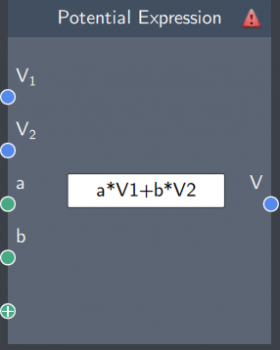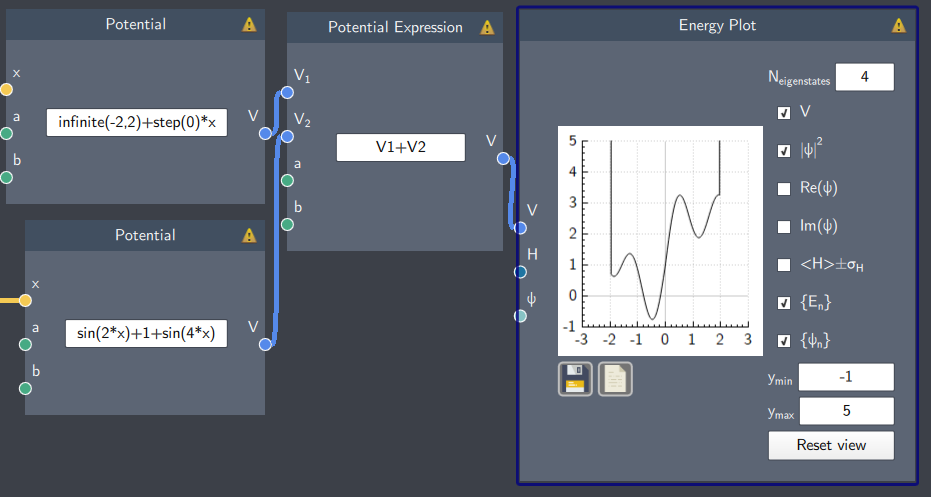# Potential Expression

## Description

In this node, a potential expression can be obtained by combining two potential functions with an arithmetic operation.## Inputs

The node has the following inputs:

• Potential functions (V$_{1}$ and V$_{2}$):  It inputs two Potential nodes (with defined functions in these nodes).
• Scalars ($a$, $b$ etc.) - It is possible to input scalar quantities to parameterize the expression.

## Content

In the content field, an arithmetic operation is defined to combine the two potential functions entered in the Potential nodes.

## Output

After all the inputs are provided, the node gives the following output:

• Potential (V) : The resulting potential function combining the input potential functions and the arithmetic operation.

## Example

An example is shown below where two potential functions are added together to plot the resulting potential.

This allows the user to visualize the system and ensure the potential's correctness if the final potential function is a long combination of many functions.